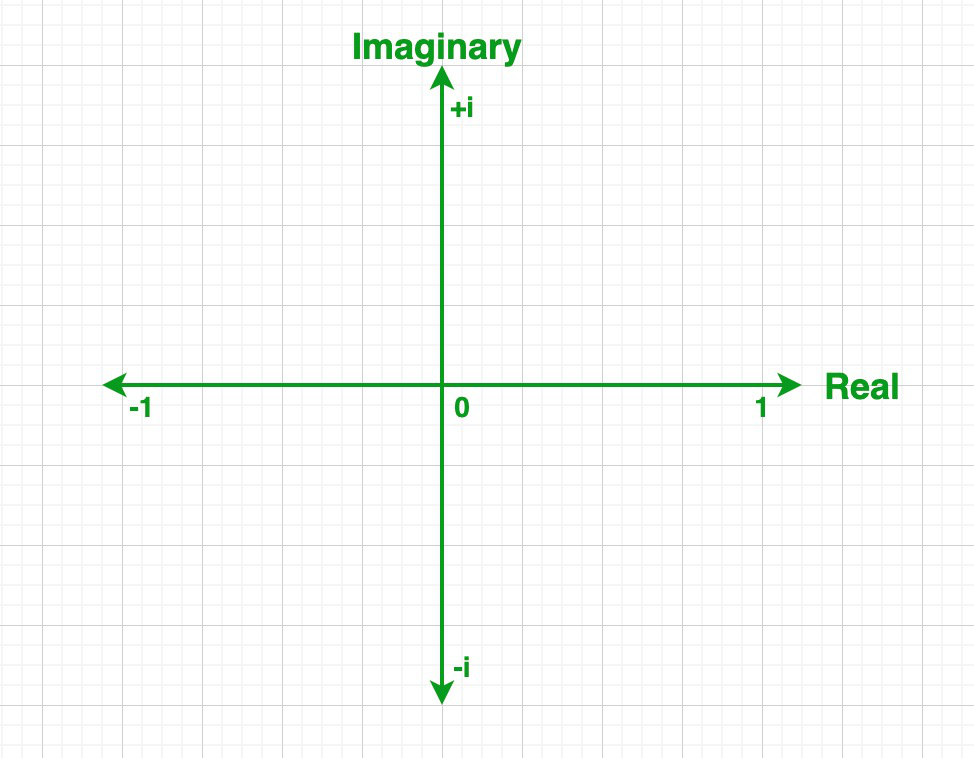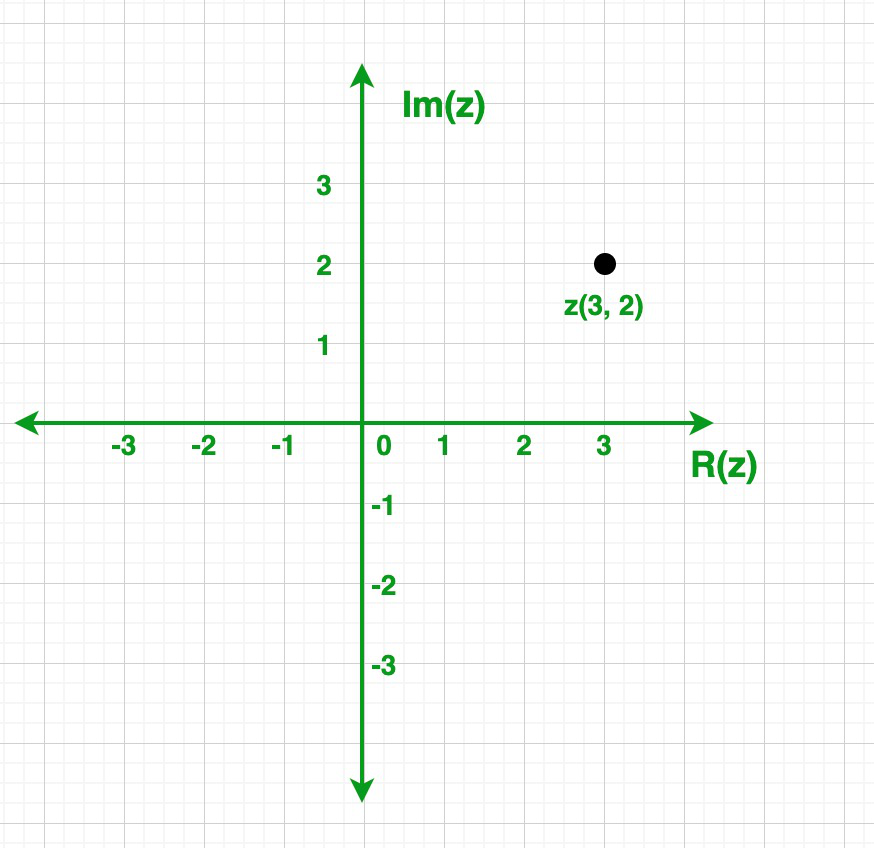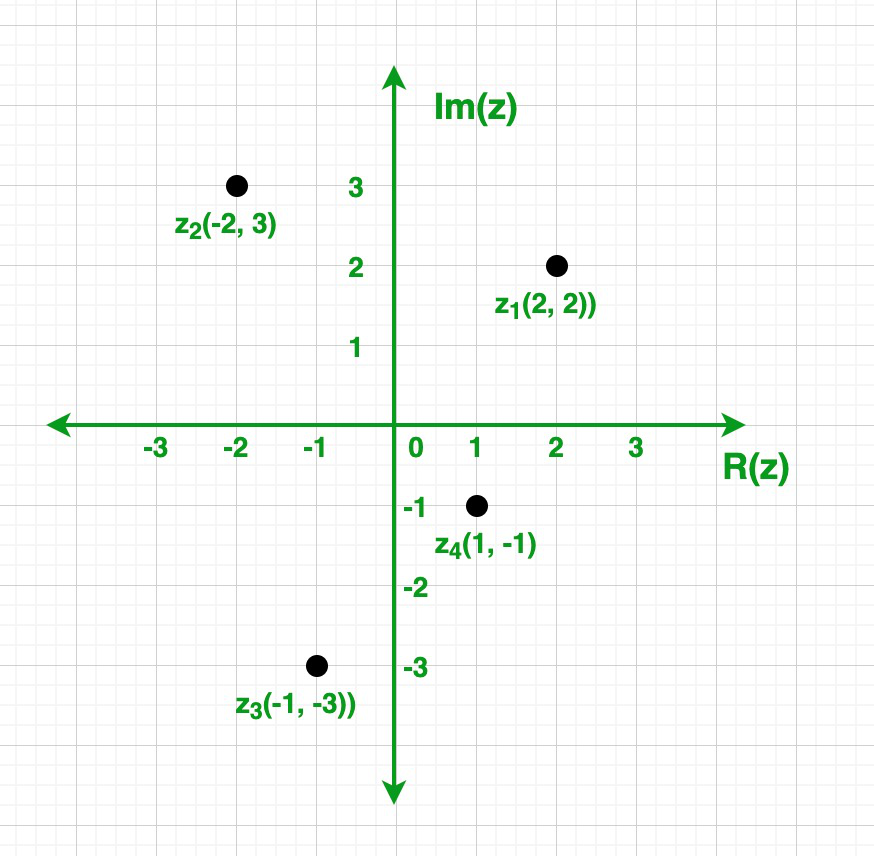Open in App
Not now

## Related Articles

• CBSE Class 11 Syllabus
• CBSE Class 11 Maths Notes
• CBSE Class 11 Physics Notes
• CBSE Class 11 Biology Notes
• CBSE Class 11 | Computer Science – C++ Syllabus

# Complex Numbers

• Difficulty Level : Easy
• Last Updated : 12 Mar, 2021

Complex Numbers are the numbers of the form (a + i b) where a & b are the real numbers and i is an imaginary unit called iota that represents √-1. For example, 2 + 3i, is a complex number in which 2 is a real number and 3i is an imaginary number.

### Need for Complex Numbers

Initially, we only have the set of natural numbers (N) which is extended to form a set of integers (I) as the equation (x + a = b) is not solvable for a>b where a, b ∈ N.

Then this set of integers (I) is extended to a set of rational numbers (Q) as every equation of the form (x.a = b) is not uniquely solvable where a ≠ 0 and a, b ∈ I.

Again this set of integers (Q) is extended to a set of real numbers (rational & irrational) (R) as every equation of the form (x2 = a) is not solvable where a > 0 and a ∈ Q i.e x2 = 5 because there is not any rational number whose square is 5. Hence, the notion of irrational numbers is given to numbers like √2, √3, √5, etc.

Finally, we extended this set of real numbers (R) to a set of complex numbers (C) as the equation of the form (x2 = a) is not solvable where a < 0 and a ∈ R i.e x2 + 5 = 0 because there is not any real number whose square is -5. Hence, the notion of complex (imaginary) numbers is given to numbers like √-1, √-2, √-3, √-5, etc. where √-1 is called imaginary unit (iota) and represented by the symbol i (i2 = -1).

### Classification of Complex Numbers

As we know the standard form of a complex number is z = (a + i b) where a, b ∈ R, and i is iota (an imaginary unit). So depending on the values of a (called real part) and b (called imaginary part), the complex numbers are classified into four types:

1. Zero Complex Number (a = 0 & b = 0)

Example: 0 (zero)

2. Purely Real Numbers (a ≠ 0 & b = 0)

Examples: 2, 3, 7, etc.

3. Purely Imaginary Numbers (a = 0 & b ≠ 0)

Examples: -7i, -5i, -i, i, 5i, 7i, etc.

4. Imaginary Numbers (a ≠ 0 & b ≠ 0)

Examples: (-1 – i), (1 + i), (1 – i), (2 + 3i), etc.

### Different Forms of Complex Numbers

1. Rectangular Form also called Standard Form = (a + i b)

Examples: (5 + 5i), (-7i), (-3 – 4i), etc.

2. Polar Form = r [cos(θ) + i sin(θ)]

Examples: [cos(π/2) + i sin(π/2)], 5[cos(π/6) + i sin(π/6)], etc.

3. Exponential Form =Examples: ei(0), ei(π/2), 5.ei(π/6), etc.

NOTE: All three forms of the complex numbers discussed above are interconvertible.

### The Complex Plane

The plane on which the complex numbers are uniquely represented is called the Complex plane or Argand plane or Gaussian plane.

The Complex plane has two axes:

1. X-axis

• All the purely real complex numbers are uniquely represented by a point on it.
• Real part Re(z) of all complex numbers are plotted with respect to it.
• That’s why X-axis is also called Real axis.

2. Y-axis

• All the purely imaginary complex numbers are uniquely represented by a point on it.
• Imaginary part Im(z) of all complex numbers are plotted with respect to it.
• That’s why Y-axis is also called Imaginary axis.### Geometrical Representation of Complex Numbers

As we know that every complex number (z = a + i b) is represented by a unique point p(a, b) on the complex plane and every point on the complex plane represents a unique complex number.

To represent any complex number z = (a + i b) on the complex plane follow these conventions:

• Real part of z (Re(z) = a) becomes the X-coordinate of the point p
• Imaginary part of z (Im(z) = b) becomes the Y-coordinate of the point p

And finally z (a + i b) ⇒ p (a, b) which is a point on the complex plane.

Example 1: Plot these complex numbers z = 3 + 2 i on the Complex plane.

Solution:

Given:

z = 3 + 2 i

So, the point is z(3, 2). Now we plot this point on the below graph, here in this graph x-axis represents the real part and y-axis represents the imaginary part.Example 2: Plot these complex numbers z1 = (2 + 2 i), z2 = (-2 + 3 i), z3 = (-1 – 3 i), z4 = (1 – i) on the Complex plane.

Solution:

Given:

z1 = (2 + 2 i)

z2 = (-2 + 3 i)

z3 = (-1 – 3 i)

z4 = (1 – i)

So, the points are z1 (2, 2), z2(-2, 3), z3(-1, -3), and z4(1, -1). Now we plot these points on the below graph, here in this graph x-axis represents the real part and y-axis represents the imaginary part.My Personal Notes arrow_drop_up